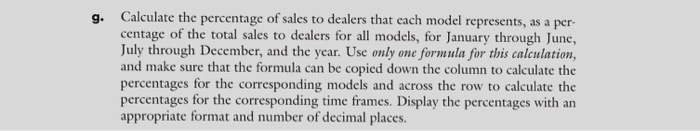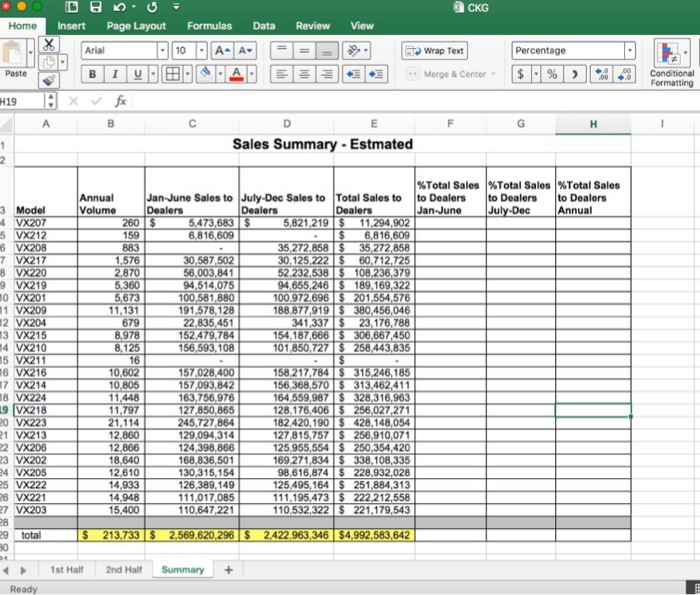### Create an Account

Home / Questions / Calculate the percentage of sales to dealers that each model represents as a per centage...

# Calculate the percentage of sales to dealers that each model represents as a per centage of the total sales to dealers for all models for January through June July through December and theg. Calculate the percentage of sales to dealers that each model represents, as a per- centage of the total sales to dealers for all models, for January through June, July through December, and the year. Use only one formula for this calculation, and make sure that the formula can be copied down the column to calculate the percentages for the corresponding models and across the row to calculate the percentages for the corresponding time frames. Display the percentages with an appropriate format and number of decimal places.

Apr 05 2020 View more View LessSubscribe To Get Solution